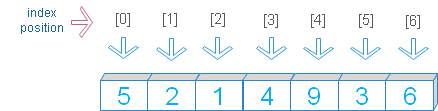# slice()

slice(start,stop,step) returns slice object for breaking string, list , tuple etc.
 start (optional) default = 0 , The start position of the slice stop ( required ), slice should Stop before this position step ( optional ) default =1 , step to consider for slicingStart, stop and step values are indices of a range.

If one value is given then it is considered as stop value as default start is 0 and step value is 1.
If two values are given then it is considered as start and stop values and step value is set to 1
If three values are given then they are considered as start , stop and step values.

## creating slice object

``````obj_slice1=slice(2)
print(obj_slice1)       # slice(None, 2, None)
obj_slice2=slice(2,4)
print(obj_slice2)       # slice(2, 4, None)
obj_slice3=slice(2,4,2)
print(obj_slice3)       # slice(2, 4, 2) ``````

## applying slice object

We will apply these slice objects to our list, tuple, string etc to break them at different positions.

Let us use slice object to break a list. While creating the slice object we have taken one parameter only so it will be used as stop value. Here start = 0 , stop=2 and step =1. Starting from 0th position, the slice will stop before 2 ( i.e at the 1st position ). The default value for step is 1.
``````obj_slice=slice(2)
my_list=[5,2,1,4,9,3,6]
print(my_list[obj_slice])  # [5,2]``````
Now start=2, stop=5 and step=1
``````obj_slice=slice(2,5,1)
my_set=(5,2,1,4,9,3,6)
print(my_set[obj_slice]) # (1,4,9)``````

## Using a string

start=2, stop=5, step=1
``````obj_slice=slice(2,5)
my_str='plus2net'
print(my_str[obj_slice]) # us2``````

## Using negative indices

Count position from right end.
``````obj_slice=slice(-5,-2)
my_str='plus2net'
print(my_str[obj_slice]) # s2n``````
``````obj_slice=slice(-7,-1,2)
my_str='plus2net'
print(my_str[obj_slice]) # lsn``````

## data type of slice()

We will use type() to get the data type of the slice object
``````obj_slice=slice(1,5,1)
print(type(obj_slice)) # <class 'slice'>``````
``````obj_slice=slice(2,5)
my_set={5,2,1,4,9,3,6}
print(my_set[obj_slice])``````
Error will be generated.

Subscribe to our YouTube Channel here

## Subscribe

* indicates required
Subscribe to plus2netplus2net.com